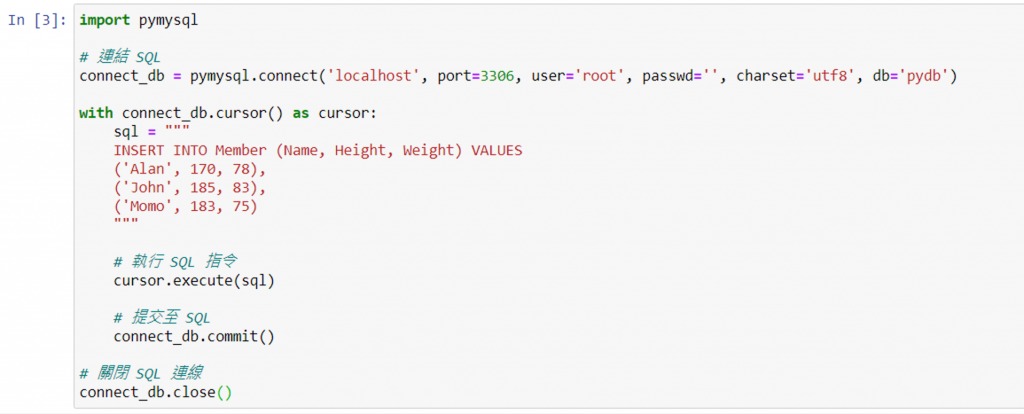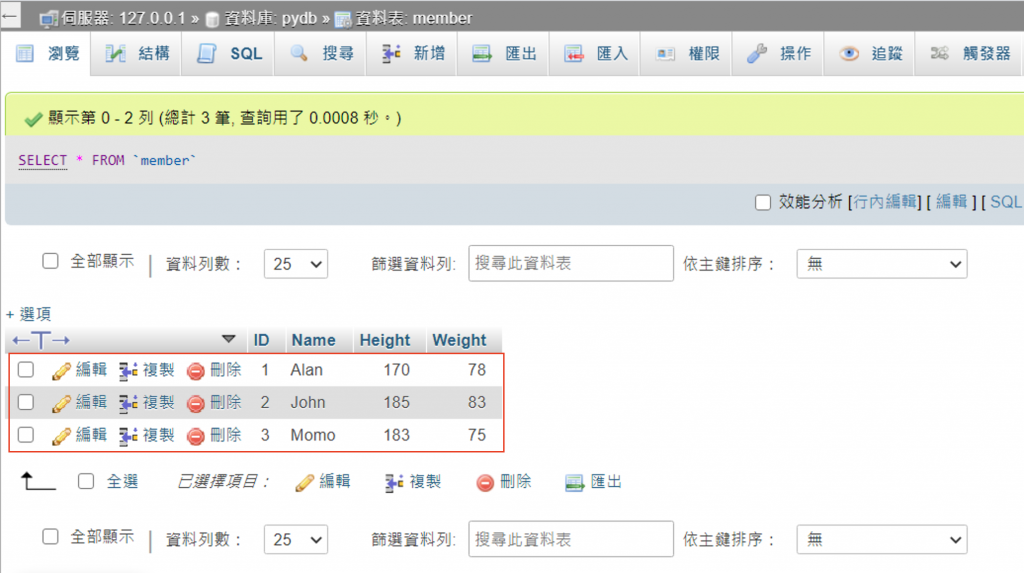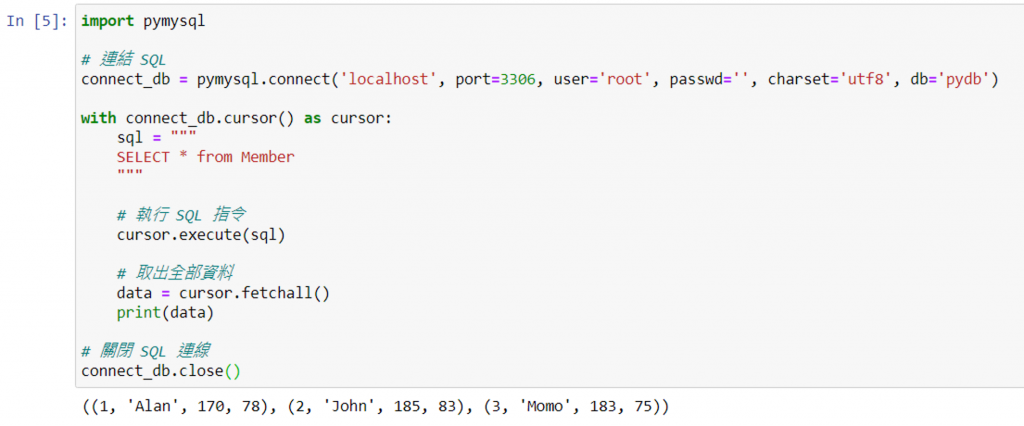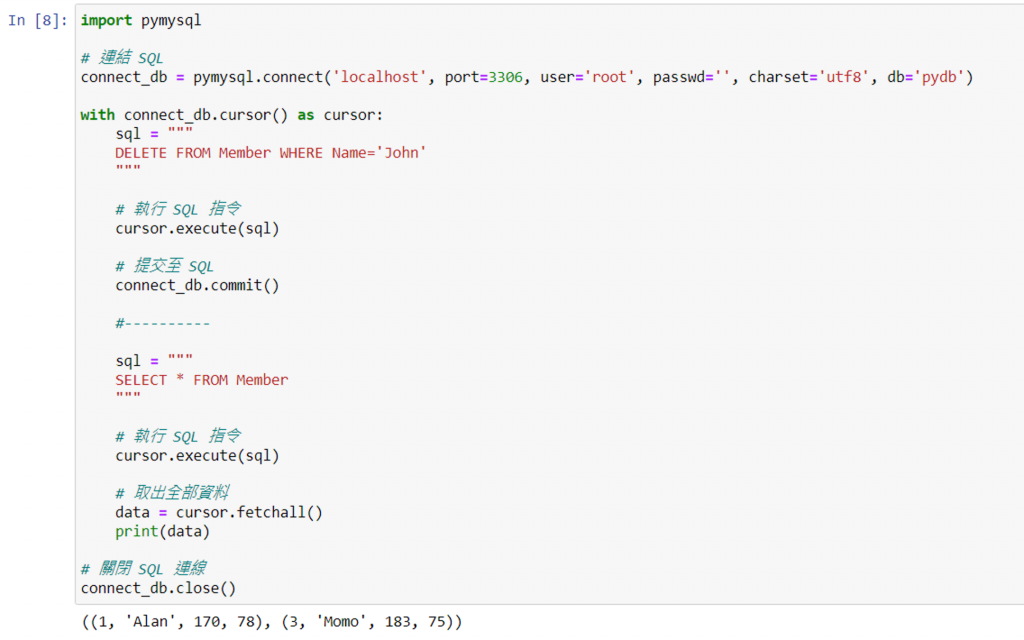#DAY 18
1
Software Development

## [2020鐵人賽Day18]糊裡糊塗Python就上手-MySQL操作探險歷程(下)

### 實際演練

#### 「新增資料」

``````sql語法變數 = """
INSERT INTO 資料表名稱 (欄位1, 欄位2, ...) VALUES (數值1, 數值2, ...), (數值1, 數值2, ...)
"""
``````

``````import pymysql

# 連結 SQL
connect_db = pymysql.connect('localhost', port=3306, user='root', passwd='', charset='utf8', db='pydb')

with connect_db.cursor() as cursor:
sql = """
INSERT INTO Member (Name, Height, Weight) VALUES
('Alan', 170, 78),
('John', 185, 83),
('Momo', 183, 75)
"""

# 執行 SQL 指令
cursor.execute(sql)

# 提交至 SQL
connect_db.commit()

# 關閉 SQL 連線
connect_db.close()
``````#### 「查詢資料」

(若是查詢所有資料，欄位的部分可用星號表示「*」)

``````sql語法變數 = """
SELECT 欄位1, 欄位2, ... FROM 資料表名稱 WHERE 條件式
"""
``````

fetchall()：取出全部資料

``````cursor.fetchall()
``````

fetchone()：取出第一筆資料

``````cursor.fetchone()
``````

``````import pymysql

# 連結 SQL
import pymysql

# 連結 SQL
connect_db = pymysql.connect('localhost', port=3306, user='root', passwd='', charset='utf8', db='pydb')

with connect_db.cursor() as cursor:
sql = """
SELECT * from Member
"""

# 執行 SQL 指令
cursor.execute(sql)

# 取出全部資料
data = cursor.fetchall()
print(data)

# 關閉 SQL 連線
connect_db.close()
``````#### 「更新資料」

``````sql語法變數 = """
UPDATE 資料表名稱 SET 欄位1=數值1, 欄位2=數值2, ... WHERE 條件式
"""
``````

``````import pymysql

# 連結 SQL
connect_db = pymysql.connect('localhost', port=3306, user='root', passwd='', charset='utf8', db='pydb')

with connect_db.cursor() as cursor:
sql = """
UPDATE Member SET Height=180 WHERE Name='John'
"""

# 執行 SQL 指令
cursor.execute(sql)

# 提交至 SQL
connect_db.commit()

#----------

sql = """
SELECT * FROM Member WHERE Name='John'
"""

# 執行 SQL 指令
cursor.execute(sql)

# 取出全部資料
data = cursor.fetchall()
print(data)

# 關閉 SQL 連線
connect_db.close()
``````#### 「刪除資料」

``````sql語法變數 = """
DELETE FROM 資料表名稱 WHERE 條件式
"""
``````

``````import pymysql

# 連結 SQL
connect_db = pymysql.connect('localhost', port=3306, user='root', passwd='', charset='utf8', db='pydb')

with connect_db.cursor() as cursor:
sql = """
DELETE FROM Member WHERE Name='John'
"""

# 執行 SQL 指令
cursor.execute(sql)

# 提交至 SQL
connect_db.commit()

#----------

sql = """
SELECT * FROM Member
"""

# 執行 SQL 指令
cursor.execute(sql)

# 取出全部資料
data = cursor.fetchall()
print(data)

# 關閉 SQL 連線
connect_db.close()
``````